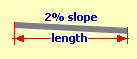Spike's Calculators

# Elevation Grade from a PercentageThis calculator will calculate the drop in inches, the difference in elevation, from a slope expressed as a percentage.

`Formula used : elevation change = ( percentage ÷ 100) * length`

##### Example calculation
a trench 20 feet long requires a 2% slope

convert 20 feet to inches
```20 * 12 = 240"
240 * (2  ÷ 100) =
240 * 0.02 =  4.8 = 4 13/16"```

### Slope Percentage Drop

Length ft in
Slope in a Percentage %
Fraction Precision #

#### Results:

 Difference in Height in

#### Calculation

1. length in feet & inches
2. required slope in a percentage
3. fraction precision preset @16

#### Result

1. required drop in inches